# Writing a Decimal and a Fraction for a Shaded Region Online Quiz

#### Complete Python Prime Pack

9 Courses     2 eBooks

#### Artificial Intelligence & Machine Learning Prime Pack

6 Courses     1 eBooks

#### Java Prime Pack

9 Courses     2 eBooks

Following quiz provides Multiple Choice Questions (MCQs) related to Writing a Decimal and a Fraction for a Shaded Region. You will have to read all the given answers and click over the correct answer. If you are not sure about the answer then you can check the answer using Show Answer button. You can use Next Quiz button to check new set of questions in the quiz.Q 1 - Write a fraction and a decimal to represent the shaded region of the figure shown below.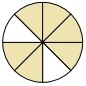### Explanation

Step 1:

The circle is divided into eight equal parts fraction of the shaded parts = $\frac{6}{8}$

Step 2:

Writing the fraction as a decimal. Using long division. $\frac{6}{8} = 0.75$

Q 2 - Write a fraction and a decimal to represent the shaded region of the figure shown below.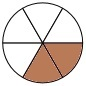### Explanation

Step 1:

The circle is divided into six equal parts fraction of the shaded parts = $\frac{2}{6}$

Step 2:

Writing the fraction as a decimal Using long division $\frac{2}{6} = 0.33…$

Q 3 - Write a fraction and a decimal to represent the shaded region of the figure shown below.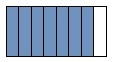### Explanation

Step 1:

The rectangle is divided into eight equal parts The fraction representing the shaded region = $\frac{7}{8}$

Step 2:

The fraction written as a decimal Using long division $\frac{7}{8} = 0.875$

Q 4 - Write a fraction and a decimal to represent the shaded region of the figure shown below.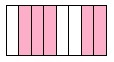### Explanation

Step 1:

The rectangle is divided into eight equal parts The fraction representing the shaded region = $\frac{5}{8}$

Step 2:

The fraction written as a decimal Using long division $\frac{5}{8} = 0.625$

Q 5 - Write a fraction and a decimal to represent the shaded region of the figure shown below.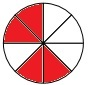### Explanation

Step 1:

The circle is divided into eight equal parts fraction of the shaded parts = $\frac{4}{8}$

Step 2:

Writing the fraction as a decimal Using long division $\frac{4}{8} = 0.5$

Q 6 - Write a fraction and a decimal to represent the shaded region of the figure shown below.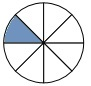### Explanation

Step 1:

The circle is divided into eight equal parts fraction of the shaded parts = $\frac{1}{8}$

Step 2:

Writing the fraction as a decimal Using long division $\frac{1}{8} = 0.125$

Q 7 - Write a fraction and a decimal to represent the shaded region of the figure shown below.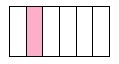### Explanation

Step 1:

The rectangle is divided into six equal parts The fraction representing the shaded region = $\frac{1}{6}$

Step 2:

The fraction written as a decimal Using long division $\frac{1}{6} = 0.167$

Q 8 - Write a fraction and a decimal to represent the shaded region of the figure shown below.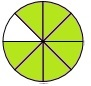### Explanation

Step 1:

The circle is divided into eight equal parts fraction of the shaded parts = $\frac{7}{8}$

Step 2:

Writing the fraction as a decimal Using long division $\frac{7}{8} = 0.875$

Q 9 - Write a fraction and a decimal to represent the shaded region of the figure shown below.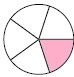### Explanation

Step 1:

The circle is divided into five equal parts fraction of the shaded parts = $\frac{1}{5}$

Step 2:

Writing the fraction as a decimal Using long division $\frac{1}{5} = 0.20$

Q 10 - Write a fraction and a decimal to represent the shaded region of the figure shown below.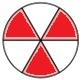### Explanation

Step 1:

The circle is divided into six equal parts fraction of the shaded parts = $\frac{3}{6}$

Step 2:

Writing the fraction as a decimal Using long division $\frac{3}{6} = 0.50$